Computer Science Level 1 - Python

Unit 5 - Detecting Walls from a Distance

# Lesson 2: Using Comparison Operators

In this Lesson, you will lean about comparison operators, and using them in a project with data from the Distance Sensor. Then you will create a project that navigates the VR Robot to the letter ‘A’ using the Distance Sensor with while loops and the greater than (>) operator. In the mini-challenge, you will apply these skills to navigate from the beginning of the Wall Maze to the number '1.'

## Learning Outcomes

• Identify that greater than (>), less than (<), and equal to (==) are comparison operators that compare two values, known as operands.
• Identify that the greater than (>) operator compares two values and reports True if the left value is larger than the right value.
• Identify that the less than (<) operator compares two values and reports True if the left value is less than the right value.
• Identify that the greater than (>) or less than (<) operators report a True or False value when used as a condition in a while loop.
• Describe how to create a project that has a VR Robot drive forward until the value of the Distance Sensor is less than a certain amount.

## Comparison Operators

The less than (<), greater than (>), and equal to (==) operators compare two values, also known as operands. The value on the left of the operator is the first operand, and the value on the right is the second operand. Operators report Boolean values and return a True value when the comparison is correct, and False when the comparison is NOT correct.

• In these examples using the less than (<) operator, when the statement is numerically correct, it will report True.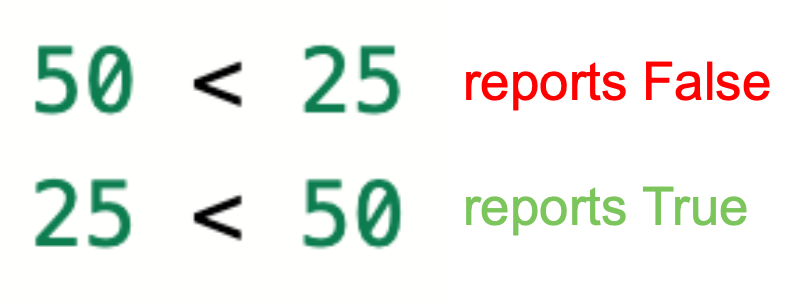• Similarly, in these examples using the greater than (>) operator, the numerically correct statement will report True.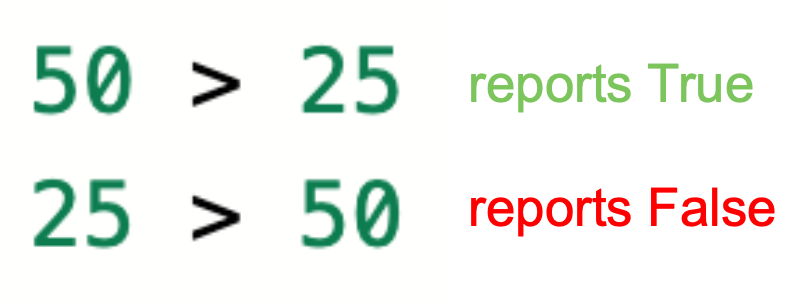• The equal to (==) operator reports True when the two values are exactly the same, and False when they are not.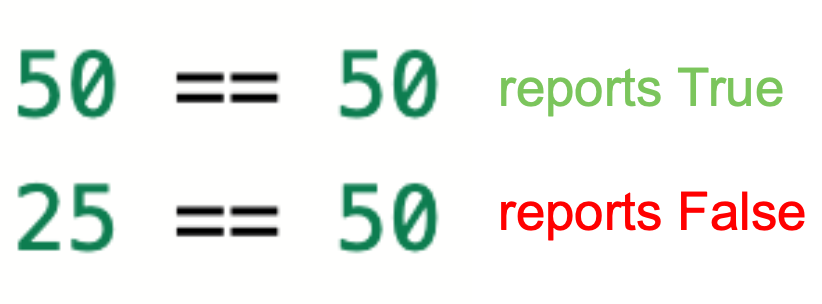• Note that the double equals sign means "equal to," and compares the two values. A single equals sign is an assignment operator that assigns a value to a variable.

Comparison operators can compare decimals, integers, or numeric reporting commands.

### Using Comparison Operators with Distance Sensor Data

Comparison operators are particularly useful when you are using numerical data from a sensor, like the Distance Sensor, to make the VR Robot make a decision. In the previous Unit, data from the Bumper Sensor was used to make the VR Robot turn when the Bumper Sensor was pressed. However, with a comparison operator, we can use data from the Distance Sensor to make the VR Robot turn at a more precise location. This is useful to avoid driving into walls, or to make the VR Robot navigate to a specific place on a Playground.

Data from the Distance Sensor can be used as one of the values in the comparison operator.For instance, if we wanted the VR Robot to drive towards an object, like a wall, then stop when it was close to the object, we could do this using a comparison operator and the get _distance function. Essentially, we are asking the VR Robot to drive while the distance between the robot and the object is greater than a threshold value, like 50 millimeters (mm).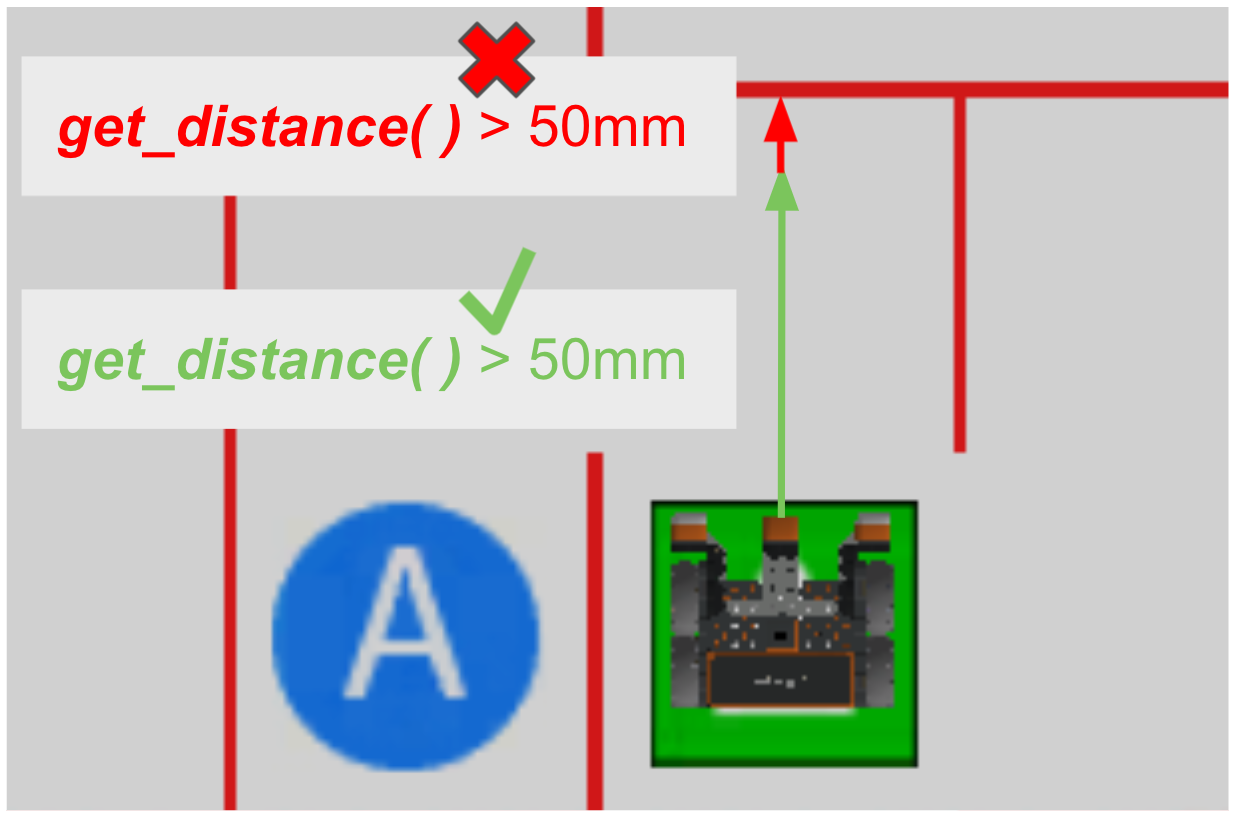While the Distance Sensor reports that it is further than 50 millimeters (mm), or the value is greater than (>) 50, the VR Robot can freely drive. When the Distance Sensor reports that it is closer than 50 millimeters (mm), or the value is not greater than (>) 50, the VR Robot needs to stop or turn before colliding with the wall.

Choosing which comparison operator to use can be a stylistic choice. The greater than (>) operator was used in the example above to make the project as clear and readable as possible. While the VR Robot is at a distance greater than 50 millimeters, drive forward. You could accomplish the same goal by reversing the order of the values in the operator, and by using a less than (<) operator.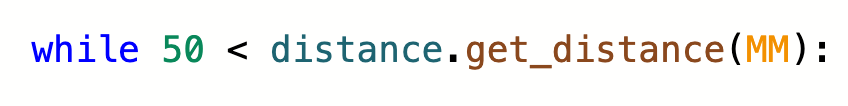Here, the VR Robot would drive while 50 millimeters was greater than the value reported by the Distance Sensor. The VR Robot would perform the same behavior, however, the project is slightly less clear and readable.

Depending on the sensor data that you are using, the equal to (==) operator may not make the VR Robot perform as you intended it to. For instance, if the goal is to have the VR Robot stop 50 millimeters from an object, you may think that of using the equal to (==) operator.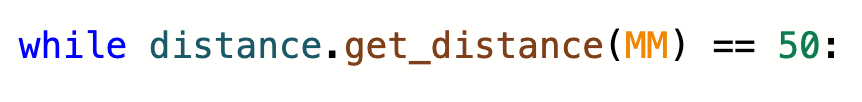However, in the Wall Maze for example, using an equal to (==) operator with the Distance Sensor would require such a precise reading from the sensor, to be exactly 50 millimeters, that the speed of the VR Robot would make it nearly impossible to get that level of precision in real time. Thus, the VR Robot would likely continue driving past the threshold because whether it is reporting a value of 100 millimeters or 25 millimeters, neither of those is equal to 50 mm, so the operator never reports as True.

### Comparison Operators and the Wall Maze Problem

A while loop can be used with comparison operators and the Distance Sensor, so that the VR Robot continually checks the sensor data and evaluates the comparison. This can enable the VR Robot to perform a behavior while a value is above or below a certain threshold. In the Wall Maze, the desired outcome is to have the VR Robot drive forward while the Distance Sensor is greater than 50 millimeters (mm) from the wall. Then, it should stop or turn to continue through the maze.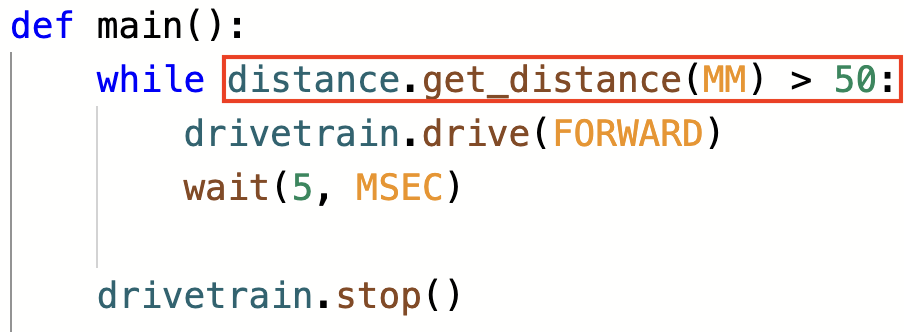In this example, the while loop will keep the VR Robot driving forward as long as the condition is met, and the distance between the VR Robot and the nearest object is reported as greater than 50 millimeters (mm).

Video file

This image explains the flow of the project above:

• While the Distance Sensor is greater than 50 millimeters (mm) from the wall, the operator reports True and stays in the while loop
• When it is less than 50 millimeters (mm) from the wall, the operator reports False, and exits the while loop.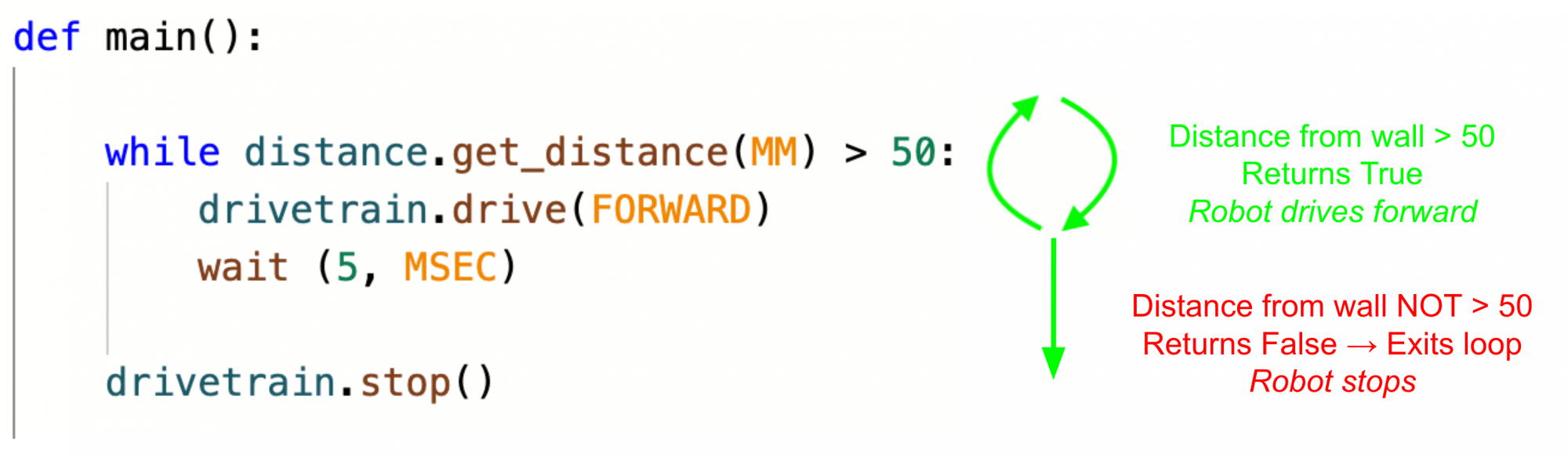Select the Next button to continue with the rest of this Lesson.